### IMO Shortlist 2011 problem N8

Kvaliteta:
Avg: 0,0
Težina:
Avg: 9,0
Let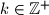$k \in \mathbb{Z}^+$ and set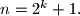$n=2^k+1.$ Prove that$n$ is a prime number if and only if the following holds: there is a permutation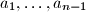$a_{1},\ldots,a_{n-1}$ of the numbers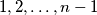$1,2, \ldots, n-1$ and a sequence of integers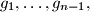$g_{1},\ldots,g_{n-1},$ such that$n$ divides$g^{a_i}_i - a_{i+1}$ for every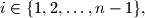$i \in \{1,2,\ldots,n-1\},$ where we set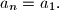$a_n = a_1.$

Proposed by Vasily Astakhov, Russia
Izvor: Međunarodna matematička olimpijada, shortlist 2011